# Analytics Calculations: Multi-Tiered Sorting

Moderator, Lightspeed Staff Posts: 66 moderator
edited June 2018
So, as we discussed earlier, one of the limitations of Analytics is that you can only sort by one Dimension, Measure or Calculation at a time.

If I want to sort by multiple tiers then, what I need is a singular column that references both values, perhaps in a creative way.

Let's say that I want to see top categories by month over the past five months...

If i sort by Month, I lose my sorting by dollar sorting...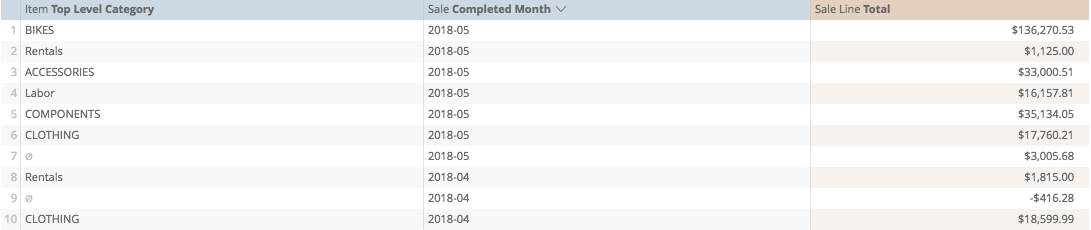If I sort by dollar, I lose my month sorting...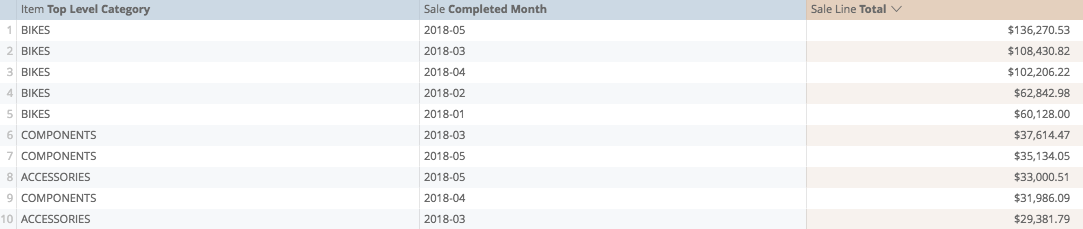What I need is a value that will sort the months first and then sort the dollar amounts within those months.

This is what we do.

The first thing we want to do, is turn the year and the month into its own number, (if we only extracted the month, then if our results took place between October and February, the sorting would be off)

From our date functions, we can create two calculations, one to extract the the year from the completed month...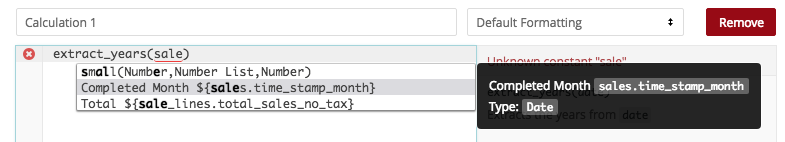...and a second to extract the month from the completed month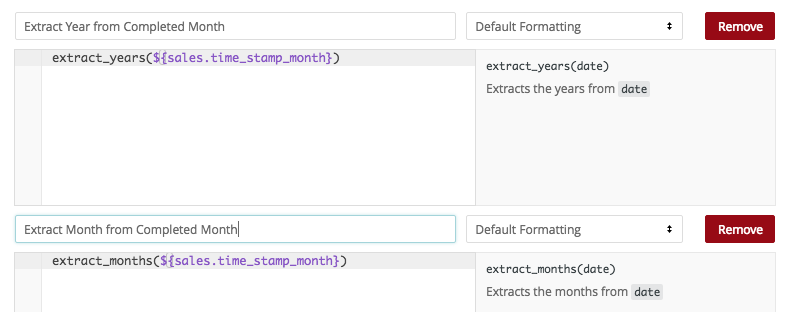So far, this is nothing remarkable, when I save, it will show me a number for the year and number for the month...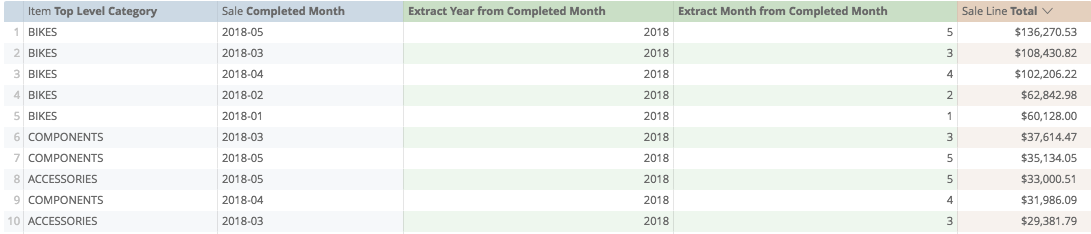The next thing we'll do is make the year and the month one number, and we'll do this by adding the two.

What I'm going to do first, is multiply the year by 100, so that 2018 becomes 201,800. This way, January becomes 201,801. December becomes 201,812. Last December becomes 201,712...

To start, following our best practices, I'm just going to add brackets to hold the place for my calculations above, one extracting the year, one extracting the month...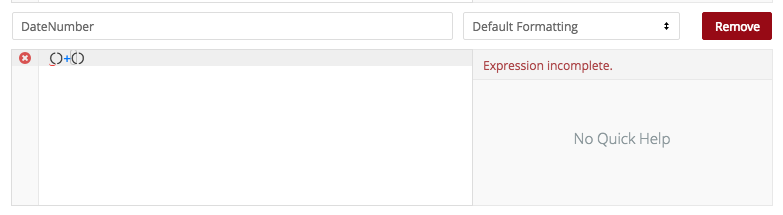I'm going to multiply the first calculation by 100, so I'm going to add that into the brackets first...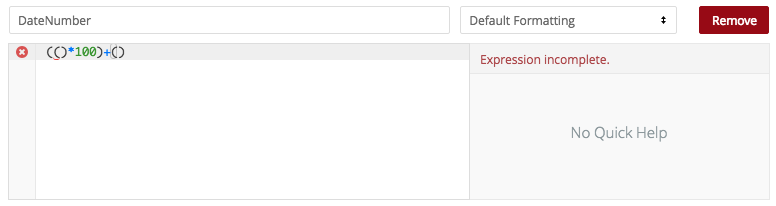Then we'll select the content from our Extract Year calculation above...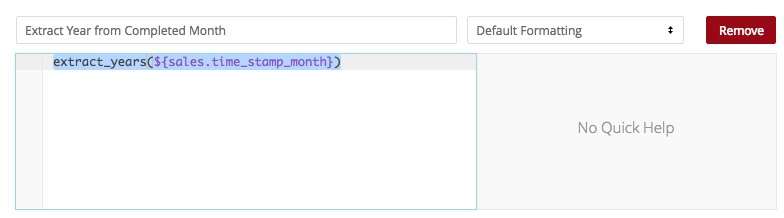and paste it between the first brackets...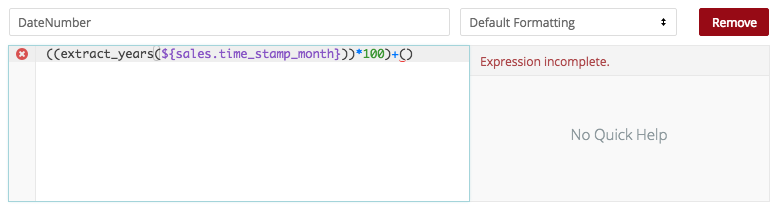then I will copy the Extract Months calculation from above...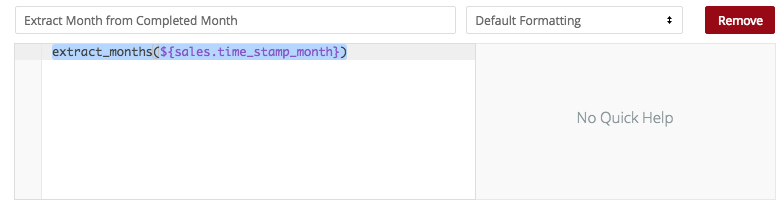..and paste it into the second set of brackets...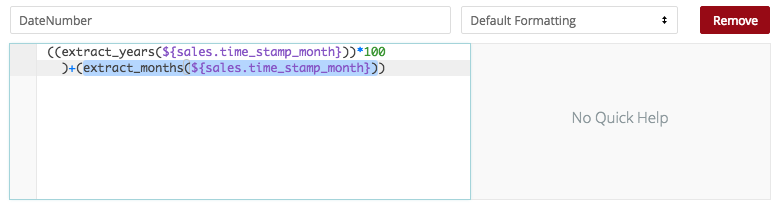Now this will look a bit funny, but when I save, I will get one number for the year and date...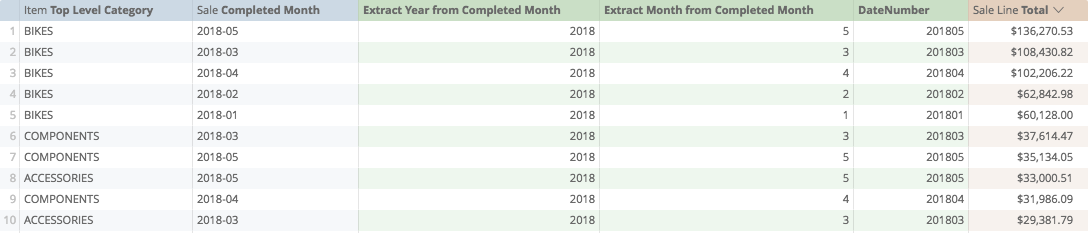Again, following our best practices, this is a good time to save version one of this report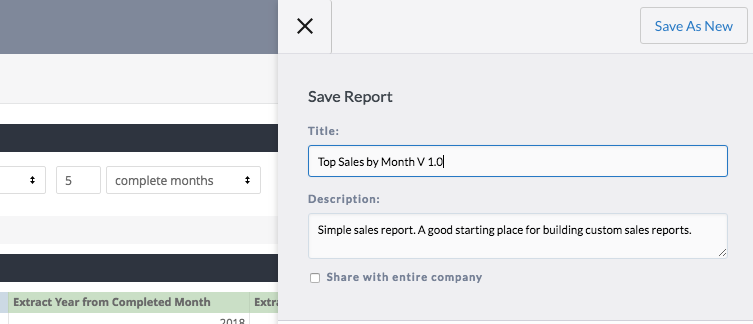Once we've Saved as new, we can begin the next step

The next thing we'll do is get the date number ready to absorb the dollar number. Our dollar numbers get to be about six digits long, so to make space, let's multiply the date by eight digits, or 10,000,000.

Again, we'll start by building the base of our calculation around brackets...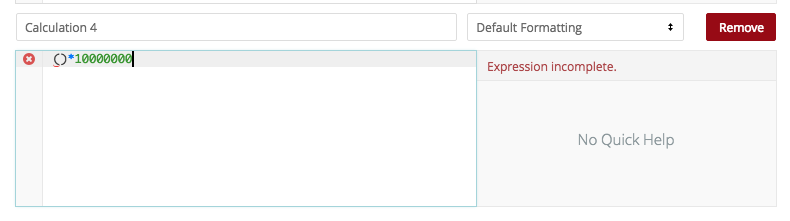Now, we'll copy the compound calculation from above...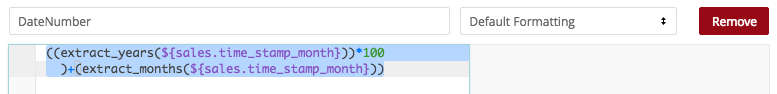...and paste it into the new one...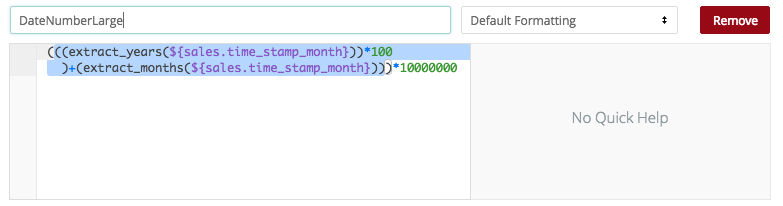...so that when we save, we get a similar number, just multiplied by 10,000,000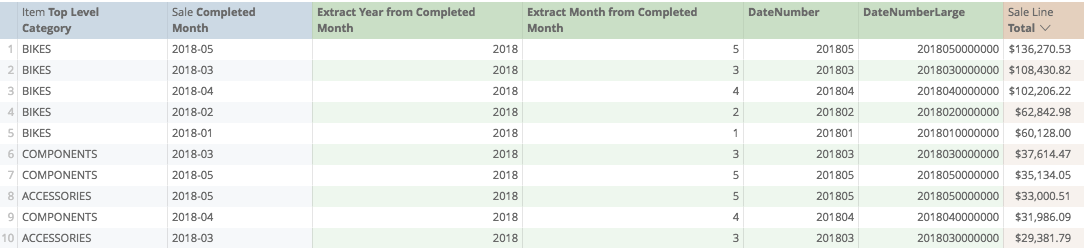...and again, this is a good time to save a new version of this report. Remember to 'Save as New", not to Save ChangesNow we get crazy. We're going to create one final calculation, including the dollar amount in the date amount...

First I start my calculation with brackets...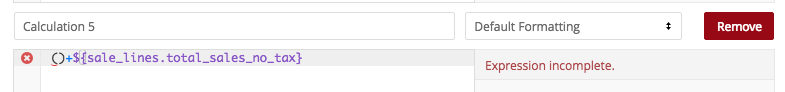Then we copy the calculation prior...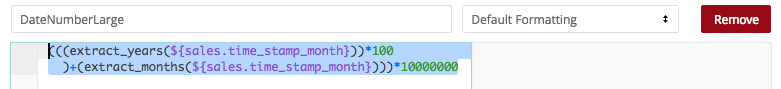...and paste it in between the brackets below...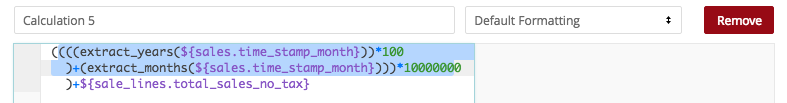Now, when I save we have one number valuing, in sequence; the year, the month, and the sale value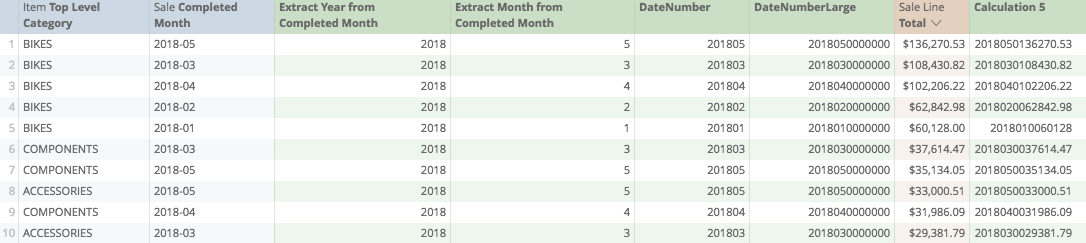Just to make sure it works, let's now sort by our last calculation...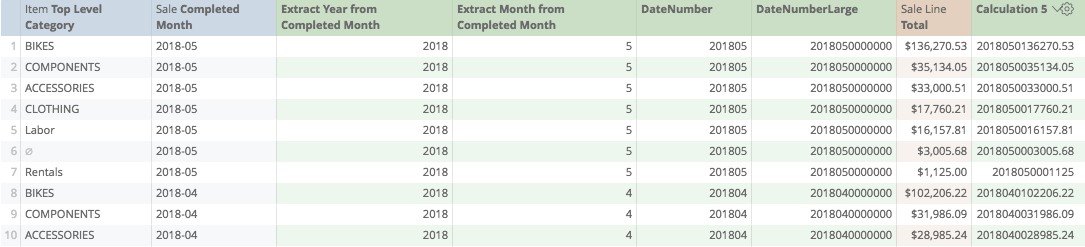And we can see now, the values sort the dollars within the month, then sort the values within the previous month.

Let's save this as a new version...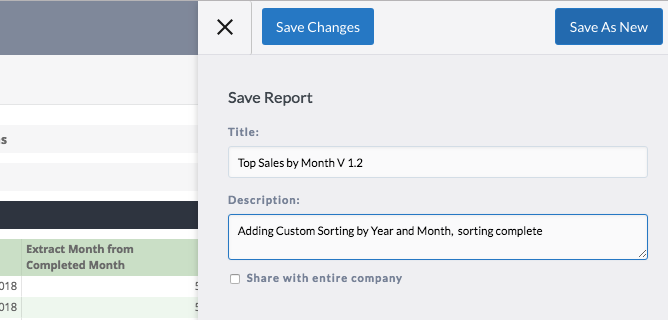Now finally, let's get rid of all the starter calculations that we don't need any more...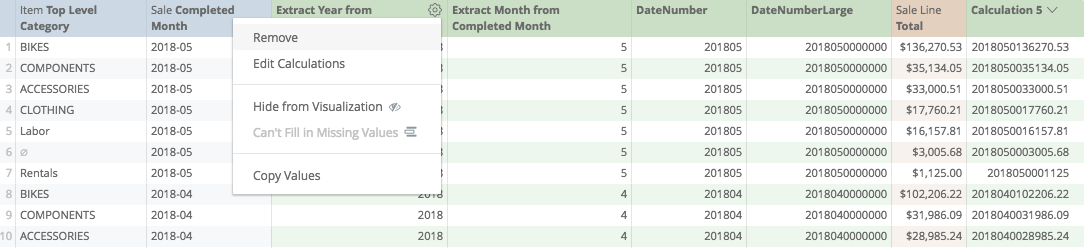Our calculations are all implicit, so we can remove one without it breaking the others...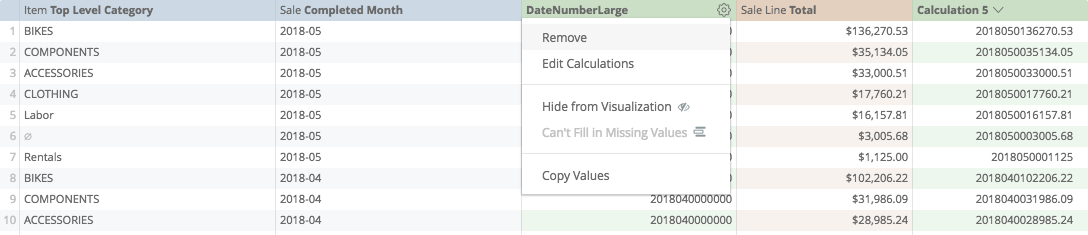and when only the last one remains (I've re-named it now), we just need to hide it from visualization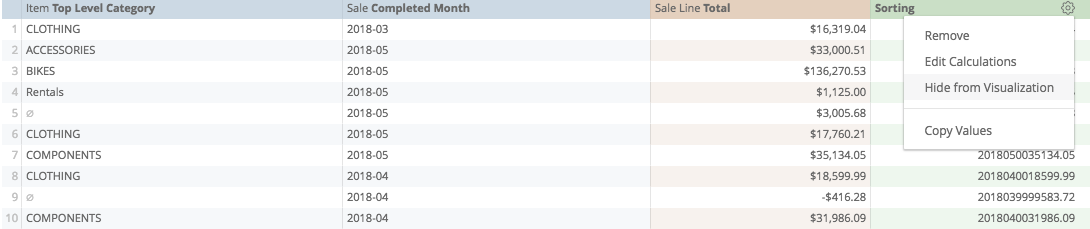So while my report includes the calculation for sorting, the final report being that I receive via email, does not.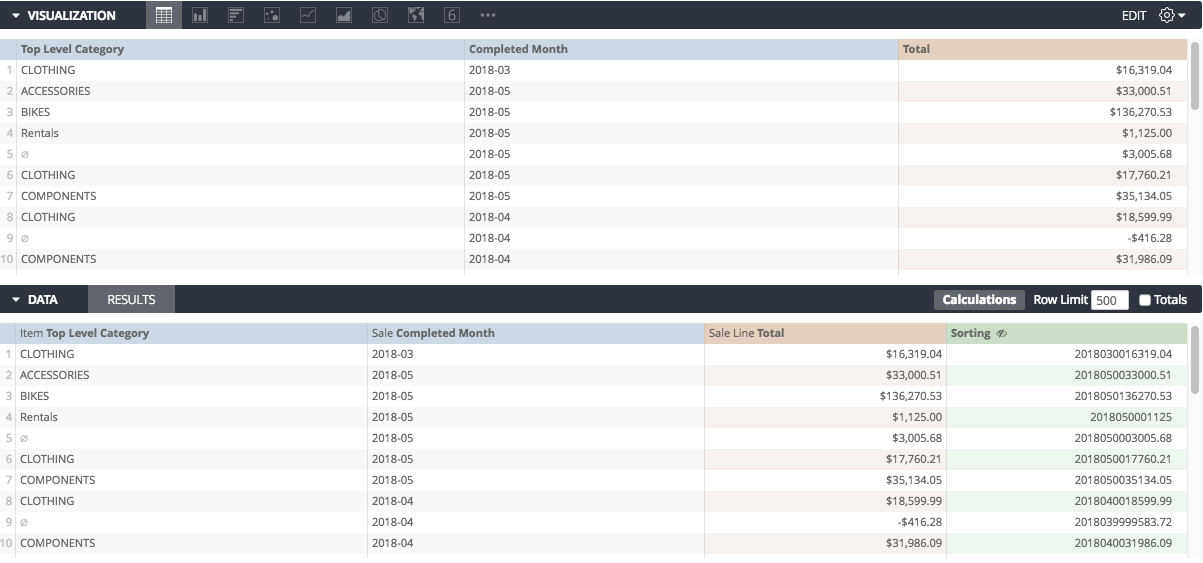The final calculation used here is:

((((extract_years(\${sales.time_stamp_month}))*100)+(extract_months(\${sales.time_stamp_month})))*10000000)+\${sale_lines.total_sales_no_tax}

Calculations Home
Joseph McKeown
Lightspeed Analytics and reporting consultant
Lightspeed HQ